×#### Thank you for registering.

One of our academic counsellors will contact you within 1 working day.

Click to Chat

1800-1023-196

+91-120-4616500

CART 0

• 0

MY CART (5)

Use Coupon: CART20 and get 20% off on all online Study Material

ITEM
DETAILS
MRP
DISCOUNT
FINAL PRICE
Total Price: Rs.

There are no items in this cart.
Continue Shopping• Complete JEE Main/Advanced Course and Test Series
• OFFERED PRICE: Rs. 15,900
• View Details

```Revision Notes on Practical Geometry

Geometry

Geometry tells us about the lines, angles, shapes and practical geometry is about the construction of these shapes.Tools to be used for construction

1. The Ruler

A ruler is a straight edge which we sometimes call a scale. It is marked with the centimetres on one side and inches on the other side. It is used to draw line segments and also to measure them.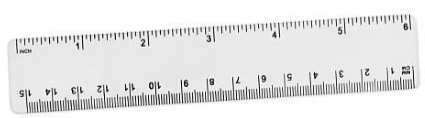2. The Compass

The compass has two ends –one is a pointer and the other is for the pencil. It is used to draw arcs and circles and also to measure the line segment.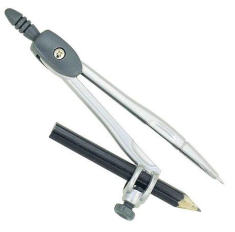3. The Divider

It is a pair of pointers on both the ends. It is used to compare the length of line and arcs.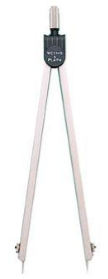4. Set -squares

It is a set of two triangular pieces.

One is having 45°, 45° and 90° angles at the corners. If we join two same triangular pieces, we will get a square. It is used to draw parallel and perpendicular lines.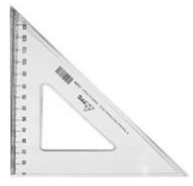Other is having 30°, 60° and 90° angles at the corners. If we join two same triangular pieces, we will get a rectangle. It is also used to draw parallel and perpendicular lines.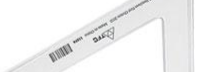5. The Protractor

It is a semi-circular device which is divided into 180-degree parts. It starts from the 0° from the right-hand side and ends with the 180° on the left-hand side and vice versa. It is used to measure and draw angles.The Circle

It is a round shape in which all the points of its boundary are at equal distance from its centre.Construction of a circle if the radius is known

Draw a circle of radius 5 cm.

To draw a circle, we need a compass and a ruler to measure the length.

Step 1: Open the compass and measure the length of 5 cm using a ruler.

Step 2: Mark a point O, which we will use as the centre of the circle.

Step 3: Put the pointer on the point O.

Step 4: Turn the compass to make a complete circle. Remember to do it in one instance.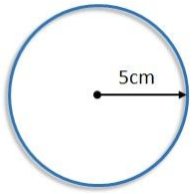A Line Segment

A line segment is a part of a line which has two endpoints. It has a fixed length so it can be measured using a ruler.

1. Construction of line segment if the length is known

Draw a line segment of 5 cm.

To draw a line segment of a particular length, we need a compass and a ruler.

Step 1: First of all draw a line l of any length and mark a point named A on it.

Step 2: Measure the length of 5 cm by putting the compass on the ruler. Put the pointer on 0 and open the compass till 5 cm on the ruler.

Step 3: Put the pointer at point A on the line and draw an arc of 5 cm which intersects the line at B.

Step 4: Hence the line segment of 5 cm is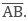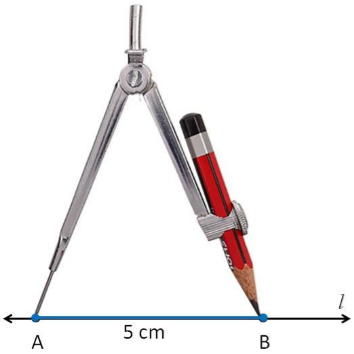2. Construction of a copy of a given line segment

To make a copy of a line segment, there are three methods-

1. Measure the length of the given line segment using a ruler and draw the line segment of the same length with the ruler only.

2. Take a transparent sheet and trace the given line on another part of the paper.

3. These methods are not very accurate so we can use a ruler and compass to make an accurate line segment.

Draw a copy of the line segmentStep 1: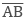is the given line segment whose length is known.Step 2: Use the compass and put the pointer on A. open the compass to place the pencil side on point B. Now the open compass is of the same length as given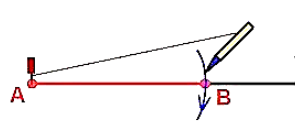Step 3: Draw a new line l and mark a point C on it. Put the pointer on the point C.

Step 4: Using the same radius draw an arc on line l which cuts the line at the point D.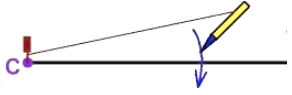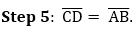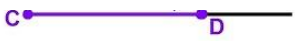Perpendiculars

If two lines intersect in such a way that they make a right angle at the point of intersection then they are perpendicular to each other.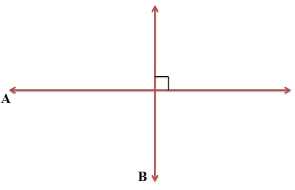1. Perpendicular to a line if a point is given on it (using a ruler and a set square)

Step 1: A line l is given and a point P on it.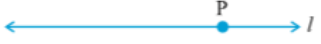Step 2: Put the ruler with one of its side next to l.Step 3: Put the set square with one of its sides by the side of the already aligned edge of the ruler so that the right-angled corner comes in contact with the ruler.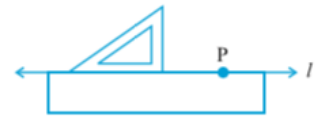Step 4:  Slide the set-square so that the right-angled corner coincides with P.Step 5: Hold the set-square. Draw PQ along the edge of the set-square.

PQ is the required perpendicular to l from the given point P.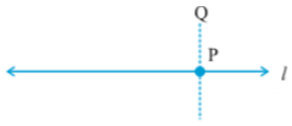2. Perpendicular to a line if a point is given on it(using a ruler and compass)

Draw a perpendicular of the line at a given point A.

Step 1: Take A as the centre and draw a big arc with any radius so that it intersects the line at point B and C.Step 2: Take B as the centre and draw an arc with the radius more than AC and then take C as center and draw an arc so that they intersect each other at point D.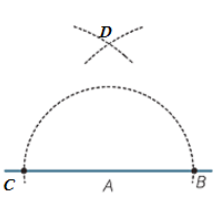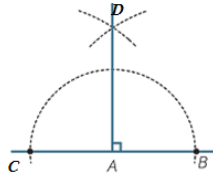3. Perpendicular to a line from a point which is not on it(using ruler and a set square)

Step 1: P is the point outside the given line l.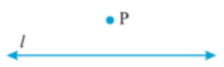Step 2: Put the set-square on l so that one side of its right angle comes on l.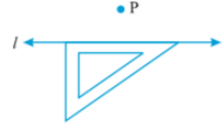Step 3: Put a ruler on the hypotenuse of set square.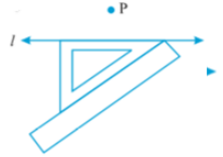Step 4: Hold the ruler and slide the set-square until it touches the point P.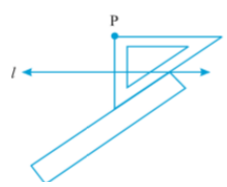Step 5: Join PM.

Now PM ⊥ l.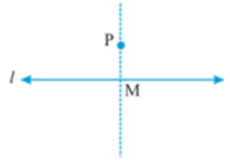4. Perpendicular to a line from a point not on it (using ruler and a compass)

Step 1: P is the point outside the given line l.Step 2: Take P as the centre and draw a big arc of any radius which intersects line l at two points A and B.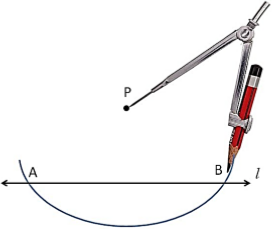Step 3: Take A and B as radius and draw arcs from both points with the same radius as taken before so that they intersect with each other.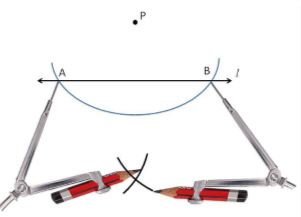Step 4: Join PQ.

PQ is the perpendicular to line l.5. The perpendicular bisector of a line segment (using transparent tapes)

A line which divides the given line into two equal parts is known as a perpendicular bisector.

Step 1: Draw a line segment AB.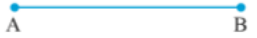Step 2: Take a strip of transparent rectangular tape and put it diagonally on the line AB so that the endpoints of the line segment lie on the edges of the tape.Step 3: Take another strip and put it over A and B diagonally across the previous tape so that the two tapes intersect at point M and N.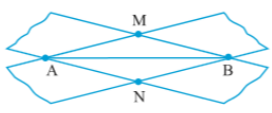Step 4: Join MN. MN is the perpendicular of AB.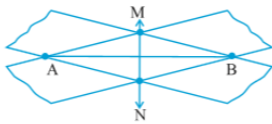6. The perpendicular bisector of a line segment (using ruler and compass)

Step 1: Draw a line segment AB.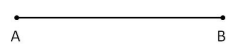Step 2: Take A as the centre and draw two arcs – one upside and one downside with the radius of more than half of the length of AB. Or you can draw a circle taking A as the centre for the convenience.

Again make the arcs with taking B as the centre so that they intersect the previous arcs.Step 3: Join the intersections of the arcs and name them as C and D.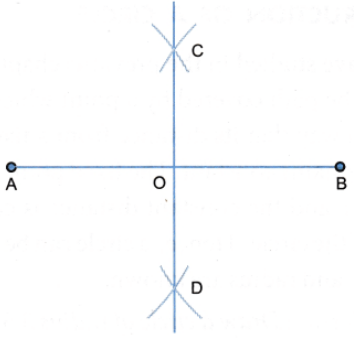Step 4: The required perpendicular bisector of AB is CD. Hence, AO = OB.

Angles

Angle is a stature formed by two rays. The two rays have a common endpoint which is called the Vertex of the Angle.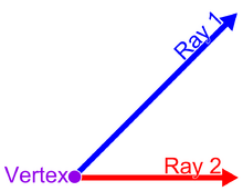1. Construction of an angle with a given measure

Draw an angle of 60 ° using a protractor.

Step 1: Draw a line BA.Step 2: Put the protractor on the line in such a way that the centre of the protractor lays on point B and the zero edge comes on the line segment BA.

Step 3: Start from 0° and mark the point C at 60°.

Step 4: Join BC.∠CBA is the required angle.

2. Construction of a copy of an angle of unknown measure (using a ruler and a compass).

Draw a copy of ∠A.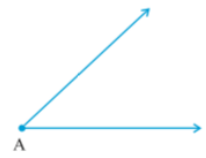Step 1: P is a point on the line l.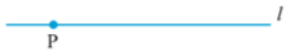Step 2: Take A as the centre in the given angle and draw an arc of any radius which cuts the two rays at B and C.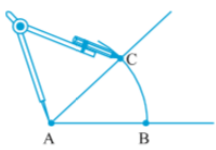Step 3: In the line l, take P as the centre and draw an arc with the same radius as above which cuts line l at Q.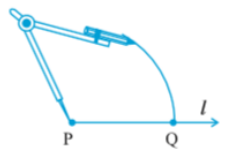Step 4: Open your compass to take the length of the arc BC.Step 5: Take Q as the centre and draw an arc with the same radius, to cut the arc drawn earlier, at point R.Step 6: Join PR. It will make the angle of the same measure as given.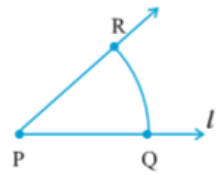Hence, ∠P = ∠A

3. The bisector of an angle

An angle bisector is the line segment which divides a particular angle into two equal parts. It is also called the line of symmetry of the angle.

Construction of angle bisector (using a ruler and a compass)

Draw the angle bisector of ∠O.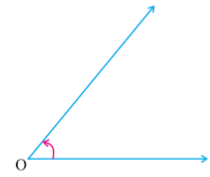Step 1: Put the pointer on O and draw an arc of any radius so that it cut the rays at point A and B.Step 2: Put the pointer on point A and draw an arc of the radius of more than half of AB.Step 3: While taking B as the centre we will draw an arc of the same radius so that it cut the previous arc at point C.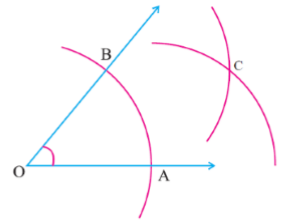Step 4: Join OC.OC is the required angle bisector of ∠O.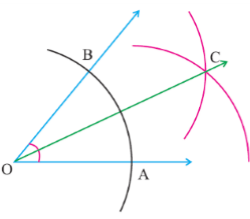Hence, ∠BOC = ∠COA.

4. Angles of special measures

There are some angles which we can construct accurately with the help of a compass without using a protractor.

a. Construction of 60° angle.

Step 1: Draw a line m and mark a point C on it.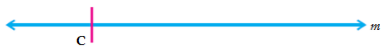Step 2: Take C as the centre and draw an arc of any radius to cut the line at point D.

Step 3: While taking D as the centre we need to draw an arc of the same radius to cut the previous arc.Step 4: Join CE. ∠C = 60°.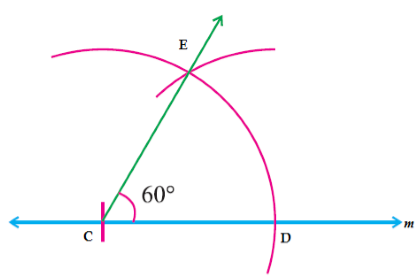b. Construction of 120° angle.

It is the twice of 60° angle.

Step 1: Draw a line m and mark a point C on it.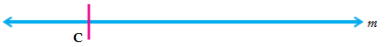Step 2: Put the pointer on point C and draw an arc of any radius which cuts the line m at point D.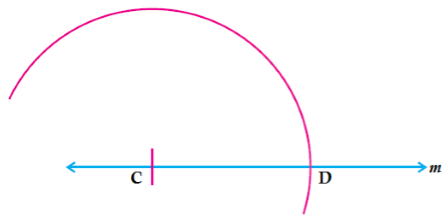Step 3: While taking D as the centre we need to draw an arc with the same radius to cut the previous arc at E.

Again take E as the centre and draw an arc of the same radius to cut the first arc at point F.Step 4: Join CF. ∠FCD = 120°.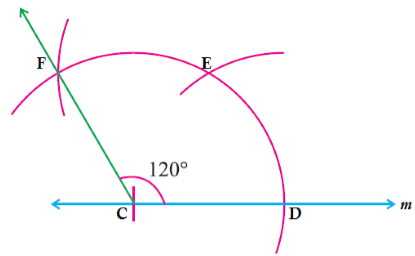c. Construction of 30° angle.

To construct an angle of 30°, we need to draw an angle of 60° as above then bisect it with the process of an angle bisector.d. Construction of 90° angle

It can be made by two methods-

i. Draw a perpendicular bisector of 180° i.e. a straight line.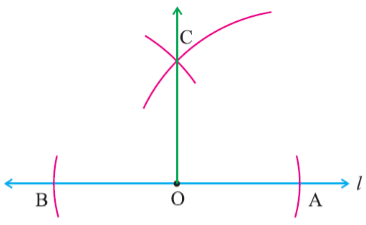ii. Draw a bisector of 60° and 120°.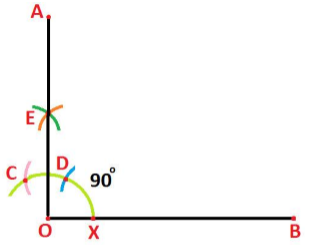e. Construction of 45° angle.

Draw an angle of 90° then bisect it to make an angle of 45°.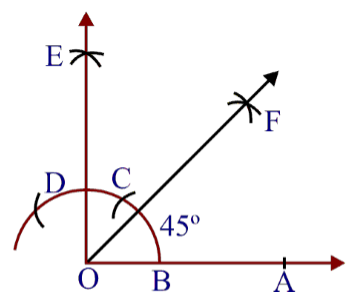```### Course Features

• 728 Video Lectures
• Revision Notes
• Previous Year Papers
• Mind Map
• Study Planner
• NCERT Solutions
• Discussion Forum
• Test paper with Video Solution